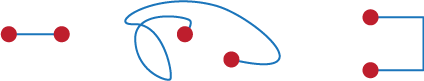## Content

### Simple graphs

The dots and line things of the last section are called graphs and from now on, we'll lift the tone of the discussion by using the more formal words vertex' for dots and edges' for lines.

Questions

1. How many graphs are there with just one vertex? The possibilities are A 0 B 1 C 547 D as many as you like. At this point you should have a discussion with your neighbour or with the whole class as to which of these is correct. When you've done that, look at the answer.
2. How many graphs are there with just two vertices1? The possibilities are A 1  B 2 C 547 D  as many as you like. Again it is time to go into camera and work with a friend. Only then look at the answer.

The answers to Questions 8 and 9 force us to make a decision on what a graph actually is. First, any edge between two vertices can be drawn any way you like. It doesn't matter for the practical purposes that graphs are used for. Whatever shape you use it's the same edge.

So we consider all of the graphs in the figure below to be the same.What's more it doesn't matter whether we put the two vertices on the Moon or one on the Moon and one on Earth, or whatever, it's the same graph.

So we have two graphs on two vertices: one with no edges and one with one. How could we possibly have infinitely many graphs on two vertices? Well who said that there was only one edge between any two vertices? After all, when we were talking about Königsberg's bridges before, we sometimes had two lines joining two vertices. Edges like this are what are called multiple edges and we can use as many multiple edges as we like in a graph. However, such graphs are called multigraphs. See https://en.wikipedia.org/wiki/Multigraph

It's worth noting that loops are considered to be multiple edges and so multigraphs include loops. Do we allow multiple edges or not? But in fact here we won't talk about multiple edges very often. Instead we'll work mainly with graphs that have no loops and no multiple edges. These are called simple graphs. It turns out then, that there are only two simple graphs with two vertices. One has an edge and the other doesn't have any.

From here on, to make things less wordy, any time we use `graph' we will mean simple graph. If we want to allow a graph to have loops or multiple edges we will specifically say so.

Questions

1. How many (simple) graphs are there with just three vertices? The possibilities are
A 2 B 3 C 4  D 8.
Before you start to think that this set of questions will go on forever, discuss Q10 with a friend. What do you need to know in order to decide this question? Then see what the answers say about it.
2. How many graphs are there with just four vertices? The possibilities are
A 8  B 9  C 10  D 11  E 12  F 21 G 22  H 23  I 24.

1The plural of vertex is vertices

Next page - Content - Isomorphism